## Some new tmux tricks (for me)

December 9, 2023 perl and general scripting hackery No comments , ,

I’ve been using tmux since RHEL stopped shipping screen by default.  We now use Oracle Linux at work, not RHEL, and perhaps Oracle Linux ships screen, but I’m unlikely to switch back now.

I blundered upon a tmux book at the Toronto Public Library today.  It’s a little book, and most of what is interesting looking to me, is in the first couple chapters.  Here are some notes.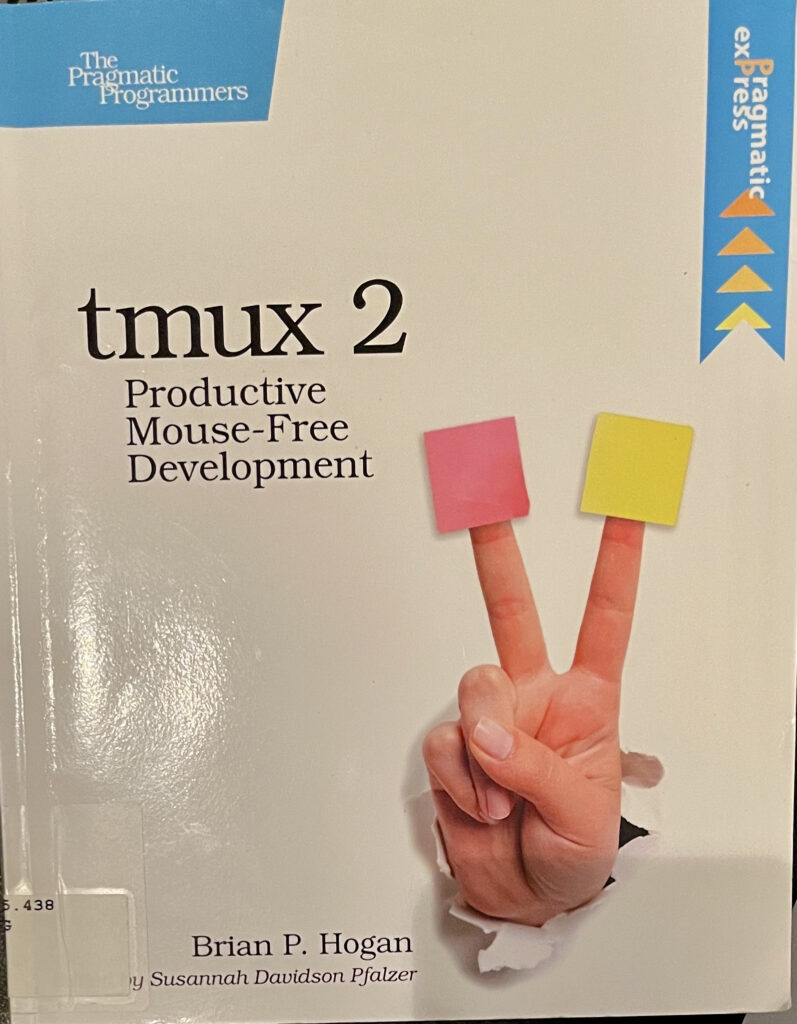## tmux sessions.

I normally want just one tmux session per machine, but tmux does have the capability of creating named sessions. I knew this, but hadn’t used it, and it’s spelled out nicely in the book.  Here are two bash aliases to exploit that feature:

alias tplay='tmux new -s play'
alias twork='tmux new -s work'


Here are two such sessions in action: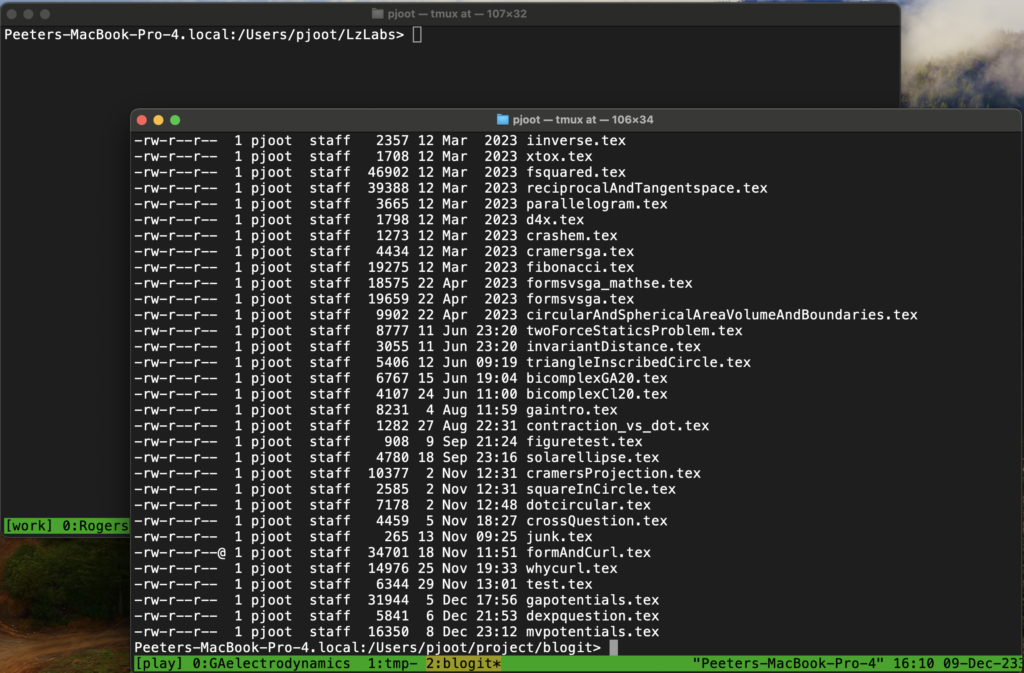Notice the little [work] and [play] indicators in the bottom left corner of each screen session.  Nice!

Of course, I created a couple aliases to attach to my named sessions:

alias atplay='tmux at -t play'
alias atwork='tmux at -t work'


The only thing screen feature that I miss is the menu of windows for the session, but I’ve gotten used to that limitation.

However, because tmux shows the active sessions at the bottom, you can use that as an built in session switching mechanism.

Do this with [Prefix] N, where N is the window number.  [Prefix] is the notation from the book that has the control sequence used for shortcuts.  For me, because I have this in my .tmux.conf

unbind C-b
set -g prefix ^N
bind n send-prefix


For me, [Prefix] is control-n (I don’t remember why I had ‘set -g’ and bind, which looks redundant to me.) So, I can do window switching with

control-n 1
control-n 2


Most of the time I use my F5, and F8 commmand key mappings to do window switching

bind-key -T root F5 select-window -p
bind-key -T root F6 select-window -l
bind-key -T root F8 select-window -n
bind-key -T root F9 new-window


but this ‘[Prefix] N’ gives me another way to do window switching.

However, what do you know, there is an equivalent to the old screen window selection mechanism, as I’ve just learned from the book:

[Prefix] w

This window switcher is actually really cool. It shows a preview of the window to be selected, and even shows the windows in both named sessions, so not only is it a window switcher, but also a session switcher!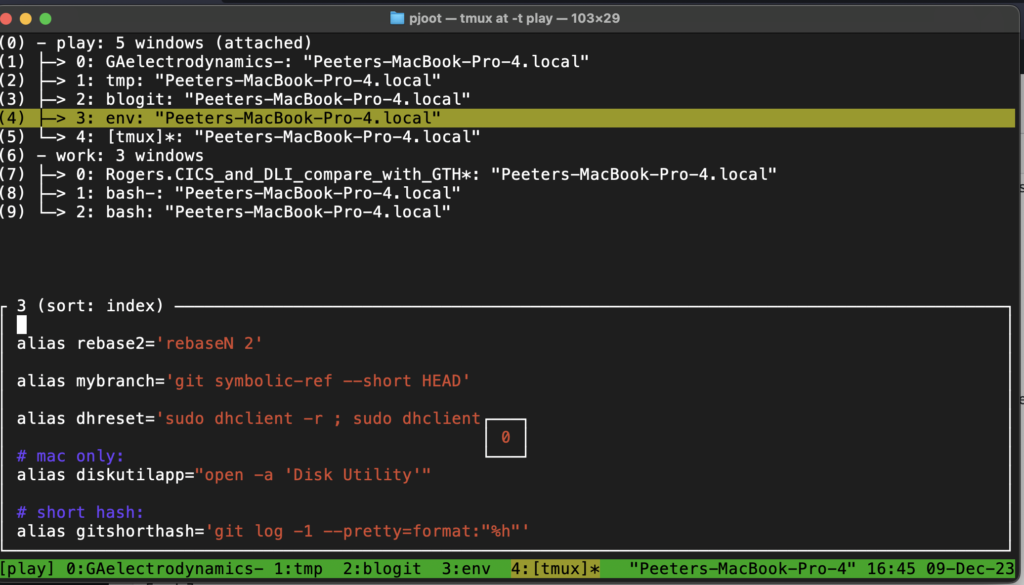## Panes

I’d seen that tmux has support for window splitting, but have never used it.

Here are the commands, for new vertical split, new horizontal split, pane cycle, and pane navigate:

[Prefix] %

[Prefix] ”

[Prefix] o

[Prefix] Up-Arrow,Down-Arrow,Left-Arrow,Right-Arrow

Looks like the pane splitting is specific to the active window, so if you do a split pane, and have run a rename-window command, for example, using something like:

alias tre='tmux rename-window'
function tnd
{
tmux rename-window pwd | sed 's,.*/,,'
}


then the split pane only shows up in that named window, like so: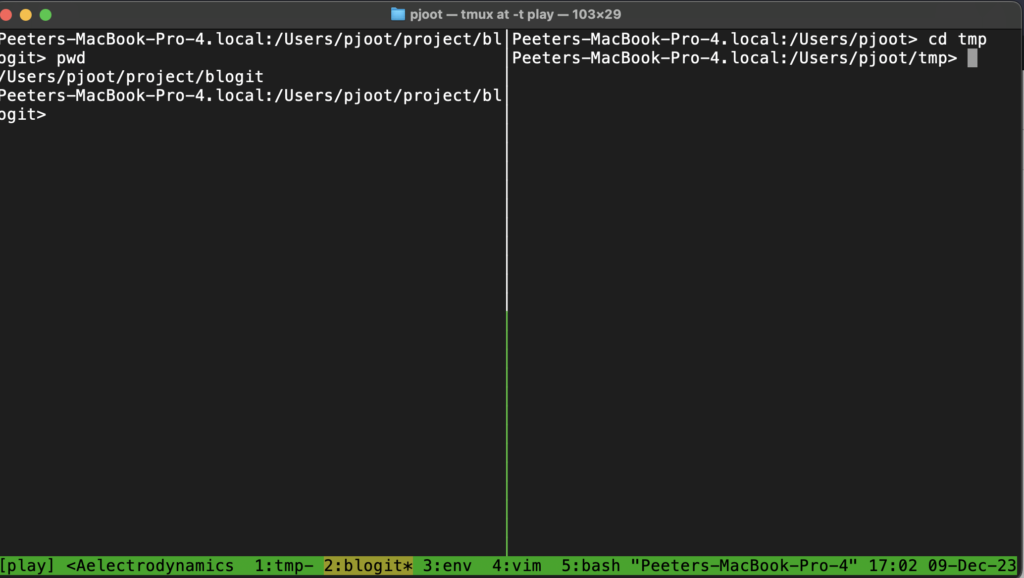## Some random tricks

Detach: [Prefix] d

(I use command line ‘tmux det’)

Switch between horizontal and vertical panes: [Prefix] space

Create new window: [Prefix] c

(I use my F9 bind-key mapping)

Pane mapping bind-keys for adjusting window size:

tmux bind -r H resize-pane -L 5
tmux bind -r J resize-pane -D 5
tmux bind -r K resize-pane -U 5
tmux bind -r L resize-pane -R 5


(can also be put into the .tmux.conf without the leading tmux)

This allows you to resize panes. For example, perhaps you want to tail the output of something long running, or run top, or something else, but have the rest of the upper pane in action, something like: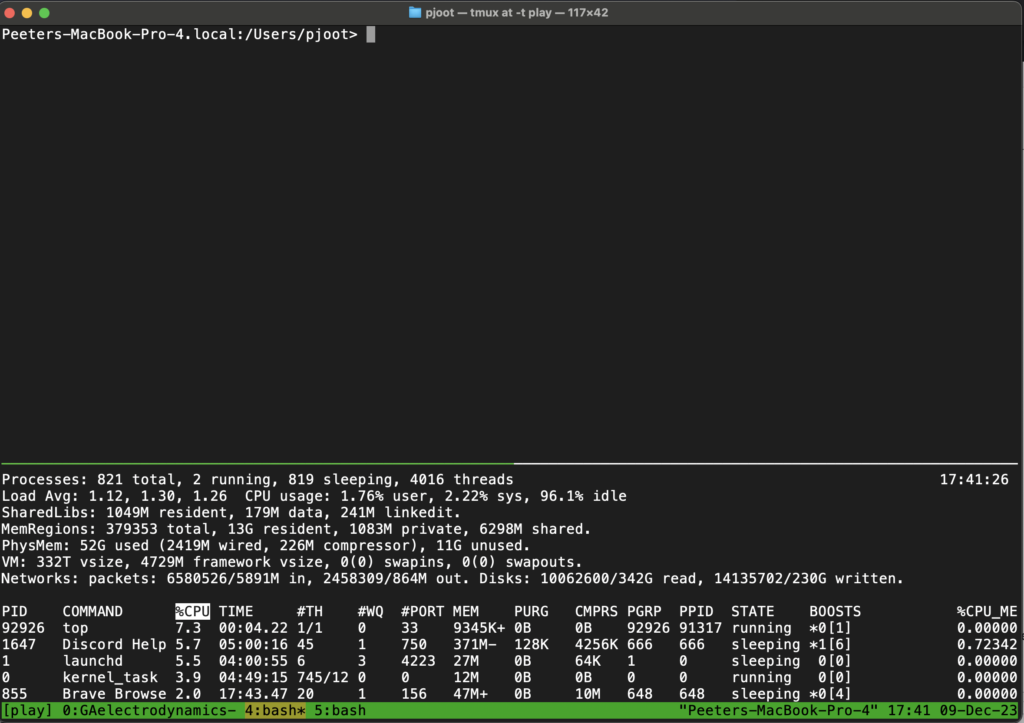## New version of Geometric Algebra for Electrical Engineers published.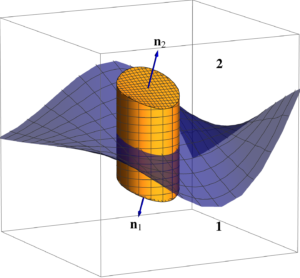A new version of my book is now published.  The free PDF and the leanpub versions are available now.  The paperback and hardcover versions should be available on Amazon within the week.

What has changed:

• V0.3.2 (Dec 8, 2023)
• Add figure showing the spherical polar conventions picked.
• Add a problem showing that $$(e^x)’ = x’ e^x$$ only when $$x$$ and $$x’$$ commute, which is true for scalars and complex numbers, but not necessarily true for abstract entities, such as multivectors and square matrices.
• Spherical polar coordinates: do not skip steps for $$\mathbf{x}_\phi$$ computation.
• Rewrite the Multivector potentials section. No longer pulling the ideas out of a magic hat, instead trying to motivate them.  Compromised on the strategy to do so, leaving some of the details to problems.

This potentials rewrite I’ve been working on indirectly for the last month, and have published two blog posts about the topic, as well another that I wrote and discarded, but helped me form and sequence some of the ideas.

The exponential derivative topic was also covered on my blog recently.  I’ve reworked that so that it is independent of the specific application to spherical polar coordinates, and set it as a problem for the reader (with solution at the end of chapter I in case I didn’t give enough hints in the problem statement.)

## Motivation.

This revisits my last blog post where I covered this content in a meandering fashion. This is an attempt to re-express this in a more compact form. In particular, in a form that is amenable to include in my book. When I wrote the potential section of my book, I cheated, and didn’t try to motivate the results. My cheat was figuring out the multivector potential representation starting with STA where things are simpler, and then translating it back to a multivector representation, instead of figuring out a reasonable way to motivate things from the foundation already laid.

I’d like to eventually have a less rushed treatment of potentials in my book, where the results are not pulled out of a magic hat. Here is an attempted step in that direction. I’ve opted to put some of the motivational material in problems (with solutions at the chapter end.)

## Multivector potentials.

We know from conventional electromagnetism (given no fictitious magnetic sources) that we can represent the six components of the electric and magnetic fields in terms of four scalar fields
\begin{equation}\label{eqn:mvpotentials:80}
\begin{aligned}
\BE &= -\spacegrad \phi – \PD{t}{\BA} \\
\BH &= \inv{\mu} \spacegrad \cross \BA.
\end{aligned}
\end{equation}
The conventional way of constructing these potentials makes use of the identities
\begin{equation}\label{eqn:mvpotentials:60}
\begin{aligned}
\end{aligned}
\end{equation}
applying those to the source free Maxwell’s equations to find representations of $$\BE, \BH$$ that automatically satisfy those equations. For that conventional analysis, see section 18-6  (available online), or section 10.1 , or section 6.4 . We can also find such a potential representation using geometric algebra methods that are cross product free (problem 1.)

For Maxwell’s equations with fictitious magnetic sources, it can be shown that a potential representation of the field
\begin{equation}\label{eqn:mvpotentials:100}
\begin{aligned}
\BH &= -\spacegrad \phi_m – \PD{t}{\BF} \\
\BE &= -\inv{\epsilon} \spacegrad \cross \BF.
\end{aligned}
\end{equation}
satisfies the source-free grades of Maxwell’s equation.
See , and  for such derivations. As with the conventional source potentials, we can also apply our geometric algebra toolbox to easily find these results (problem 2.)

We have a mix of time partials and curls that is reminiscent of Maxwell’s equation itself. It’s obvious to wonder whether there is a more coherent integrated form for the potential. This is in fact the case.

## Lemma 1.1: Multivector potentials.

For Maxwell’s equation with electric sources, the total field $$F$$ can be expressed in multivector potential form
\begin{equation}\label{eqn:mvpotentials:520}
F = \gpgrade{ \lr{ \spacegrad – \inv{c} \PD{t}{} } \lr{ -\phi + c \BA } }{1,2}.
\end{equation}
For Maxwell’s equation with only fictitious magnetic sources, the total field $$F$$ can be expressed in multivector form
\begin{equation}\label{eqn:mvpotentials:540}
F = \gpgrade{ \lr{ \spacegrad – \inv{c} \PD{t}{} } I \eta \lr{ -\phi_m + c \BF } }{1,2}.
\end{equation}

The reader should try to verify this themselves (problem 3.)

Using superposition, we can form a multivector potential that includes all grades.

## Definition 1.1: Multivector potential.

We call $$A$$, a multivector with all grades, the multivector potential, defining the total field as
\begin{equation}\label{eqn:mvpotentials:600}
\begin{aligned}
F
&=
&=
\lr{ \spacegrad – \inv{c} \PD{t}{} } A

\end{aligned}
\end{equation}
Imposition of the constraint
\begin{equation}\label{eqn:mvpotentials:680}
\end{equation}
is called the Lorentz gauge condition, and allows us to express $$F$$ in terms of the potential without any grade selection filters.

## Lemma 1.2: Conventional multivector potential.

Let
\begin{equation}\label{eqn:mvpotentials:620}
A = -\phi + c \BA + I \eta \lr{ -\phi_m + c \BF }.
\end{equation}
This results in the conventional potential representation of the electric and magnetic fields
\begin{equation}\label{eqn:mvpotentials:640}
\begin{aligned}
\BE &= -\spacegrad \phi – \PD{t}{\BA} – \inv{\epsilon} \spacegrad \cross \BF \\
\end{aligned}
\end{equation}
In terms of potentials, the Lorentz gauge condition \ref{eqn:mvpotentials:680} takes the form
\begin{equation}\label{eqn:mvpotentials:660}
\begin{aligned}
0 &= \inv{c} \PD{t}{\phi} + \spacegrad \cdot (c \BA) \\
0 &= \inv{c} \PD{t}{\phi_m} + \spacegrad \cdot (c \BF).
\end{aligned}
\end{equation}

See problem 4.

## Problem 1: Potentials for no-fictitious sources.

Starting with Maxwell’s equation with only conventional electric sources
\begin{equation}\label{eqn:mvpotentials:120}
\end{equation}
Show that this may be split by grade into three equations
\begin{equation}\label{eqn:mvpotentials:140}
\begin{aligned}
\spacegrad \wedge \BE + \inv{c}\PD{t}{} \lr{ I \eta \BH } &= 0 \\
\spacegrad \wedge \lr{ I \eta \BH } &= 0.
\end{aligned}
\end{equation}
Then use the identities $$\spacegrad \wedge \spacegrad \wedge \BA = 0$$, for vector $$\BA$$ and $$\spacegrad \wedge \spacegrad \phi = 0$$, for scalar $$\phi$$ to find the potential representation.

Taking grade(0,1) and (2,3) selections of Maxwell’s equation, we split our equations into source dependent and source free equations
\begin{equation}\label{eqn:mvpotentials:200}
\end{equation}
\begin{equation}\label{eqn:mvpotentials:220}
\end{equation}

In terms of $$F = \BE + I \eta \BH$$, the source free equation expands to
\begin{equation}\label{eqn:mvpotentials:240}
\begin{aligned}
0
&=
\lr{ \spacegrad + \inv{c} \PD{t}{} } \lr{ \BE + I \eta \BH }
}{2,3} \\
&=
&=
+ \spacegrad \wedge \lr{ I \eta \BH }
+ I \eta \inv{c} \PD{t}{\BH},
\end{aligned}
\end{equation}
which can be further split into a bivector and trivector equation
\begin{equation}\label{eqn:mvpotentials:260}
0 = \spacegrad \wedge \BE + I \eta \inv{c} \PD{t}{\BH}
\end{equation}
\begin{equation}\label{eqn:mvpotentials:280}
0 = \spacegrad \wedge \lr{ I \eta \BH }.
\end{equation}
It’s clear that we want to write the magnetic field as a (bivector) curl, so we let
\begin{equation}\label{eqn:mvpotentials:300}
I \eta \BH = I c \BB = c \spacegrad \wedge \BA,
\end{equation}
or
\begin{equation}\label{eqn:mvpotentials:301}
\BH = \inv{\mu} \spacegrad \cross \BA.
\end{equation}

\Cref{eqn:mvpotentials:260} is reduced to
\begin{equation}\label{eqn:mvpotentials:320}
\begin{aligned}
0
&= \spacegrad \wedge \BE + I \eta \inv{c} \PD{t}{\BH} \\
&= \spacegrad \wedge \BE + \inv{c} \PD{t}{} \spacegrad \wedge \lr{ c \BA } \\
&= \spacegrad \wedge \lr{ \BE + \PD{t}{\BA} }.
\end{aligned}
\end{equation}
We can now let
\begin{equation}\label{eqn:mvpotentials:340}
\BE + \PD{t}{\BA} = -\spacegrad \phi.
\end{equation}
We sneakily adjust the sign of the gradient so that the result matches the conventional representation.

## Problem 2: Potentials for fictitious sources.

Starting with Maxwell’s equation with only fictitious magnetic sources
\begin{equation}\label{eqn:mvpotentials:160}
\end{equation}
show that this may be split by grade into three equations
\begin{equation}\label{eqn:mvpotentials:180}
\begin{aligned}
-\eta \spacegrad \wedge \BH + \inv{c}\PD{t}{(I \BE)} &= 0 \\
\spacegrad \wedge \lr{ I \BE } &= 0.
\end{aligned}
\end{equation}
Then use the identities $$\spacegrad \wedge \spacegrad \wedge \BF = 0$$, for vector $$\BF$$ and $$\spacegrad \wedge \spacegrad \phi_m = 0$$, for scalar $$\phi_m$$ to find the potential representation \ref{eqn:mvpotentials:100}.

We multiply \ref{eqn:mvpotentials:160} by $$I$$ to find
\begin{equation}\label{eqn:mvpotentials:360}
\end{equation}
which can be split into
\begin{equation}\label{eqn:mvpotentials:380}
\begin{aligned}
\end{aligned}
\end{equation}
We expand the source free equation in terms of $$I F = I \BE – \eta \BH$$, to find
\begin{equation}\label{eqn:mvpotentials:400}
\begin{aligned}
0
&= \gpgrade{ \lr{ \spacegrad + \inv{c}\PD{t}{} } \lr{ I \BE – \eta \BH } }{0,3} \\
&= \spacegrad \wedge \lr{ I \BE } + \inv{c} \PD{t}{(I \BE)} – \eta \spacegrad \wedge \BH,
\end{aligned}
\end{equation}
which has the respective bivector and trivector grades
\begin{equation}\label{eqn:mvpotentials:420}
0 = \spacegrad \wedge \lr{ I \BE }
\end{equation}
\begin{equation}\label{eqn:mvpotentials:440}
0 = \inv{c} \PD{t}{(I \BE)} – \eta \spacegrad \wedge \BH.
\end{equation}
We can clearly satisfy \ref{eqn:mvpotentials:420} by setting
\begin{equation}\label{eqn:mvpotentials:460}
I \BE = -\inv{\epsilon} \spacegrad \wedge \BF,
\end{equation}
or
\begin{equation}\label{eqn:mvpotentials:461}
\BE = -\inv{\epsilon} \spacegrad \cross \BF.
\end{equation}
Here, once again, the sneaky inclusion of a constant factor $$-1/\epsilon$$ is to make the result match the conventional. Inserting this value for $$I \BE$$ into our bivector equation yields
\begin{equation}\label{eqn:mvpotentials:480}
\begin{aligned}
0
&= -\inv{\epsilon} \inv{c} \PD{t}{} (\spacegrad \wedge \BF) – \eta \spacegrad \wedge \BH \\
&= -\eta \spacegrad \wedge \lr{ \PD{t}{\BF} + \BH },
\end{aligned}
\end{equation}
so we set
\begin{equation}\label{eqn:mvpotentials:500}
\PD{t}{\BF} + \BH = -\spacegrad \phi_m,
\end{equation}
and have a field representation that automatically satisfies the source free equations.

## Problem 3: Total field in terms of potentials.

Prove lemma 1.1, either by direct expansion, or by trying to discover the multivector form of the field by construction.

Proof by expansion is straightforward, and left to the reader. We form the respective total electromagnetic fields $$F = \BE + I \eta H$$ for each case.

We find
\begin{equation}\label{eqn:mvpotentials:560}
\begin{aligned}
F
&= \BE + I \eta \BH \\
&= -\spacegrad \phi – \PD{t}{\BA} + I \frac{\eta}{\mu} \spacegrad \cross \BA \\
&= -\spacegrad \phi – \inv{c} \PD{t}{(c \BA)} + \spacegrad \wedge (c\BA) \\
&= \gpgrade{ \spacegrad \lr{ -\phi + c \BA } – \inv{c} \PD{t}{(c \BA)} }{1,2} \\
&= \gpgrade{ \lr{ \spacegrad -\inv{c} \PD{t}{} } \lr{ -\phi + c \BA } }{1,2}.
\end{aligned}
\end{equation}

For the field for the fictitious source case, we compute the result in the same way, inserting a no-op grade selection to allow us to simplify, finding
\begin{equation}\label{eqn:mvpotentials:580}
\begin{aligned}
F
&= \BE + I \eta \BH \\
&= -\inv{\epsilon} \spacegrad \cross \BF + I \eta \lr{ -\spacegrad \phi_m – \PD{t}{\BF} } \\
&= \inv{\epsilon c} I \lr{ \spacegrad \wedge (c \BF)} + I \eta \lr{ -\spacegrad \phi_m – \inv{c} \PD{t}{(c \BF)} } \\
&= I \eta \lr{ \spacegrad \wedge (c \BF) + \lr{ -\spacegrad \phi_m – \inv{c} \PD{t}{(c \BF)} } } \\
&= I \eta \gpgrade{ \spacegrad \wedge (c \BF) + \lr{ -\spacegrad \phi_m – \inv{c} \PD{t}{(c \BF)} } }{1,2} \\
&= I \eta \gpgrade{ \spacegrad (-\phi_m + c \BF) – \inv{c} \PD{t}{(c \BF)} }{1,2} \\
&= I \eta \gpgrade{ \lr{ \spacegrad -\inv{c} \PD{t}{} } (-\phi_m + c \BF) }{1,2}.
\end{aligned}
\end{equation}

## Problem 4: Fields in terms of potentials.

Prove lemma 1.2.

Let’s expand and then group by grade
\begin{equation}\label{eqn:mvpotentials:n}
\begin{aligned}
\lr{ \spacegrad – \inv{c} \PD{t}{} } A
&=
\lr{ \spacegrad – \inv{c} \PD{t}{} } \lr{ -\phi + c \BA + I \eta \lr{ -\phi_m + c \BF }} \\
&=
-\inv{c} \PD{t}{\phi} + c \inv{c} \PD{t}{ \BA } + I \eta \lr{ -\inv{c} \PD{t}{\phi_m} + c \inv{c} \PD{t}{\BF} } \\
&=
+ I \eta c \spacegrad \wedge \BF
– c \inv{c} \PD{t}{\BA}
– c I \eta \inv{c} \PD{t}{\BF} \\
+\inv{c} \PD{t}{\phi}
+ \inv{c} \PD{t}{\phi_m} } \\
&=
– \PD{t}{\BA}
– \PD{t}{\BF}
} \\
+\inv{c} \PD{t}{\phi}
+ \inv{c} \PD{t}{\phi_m} }.
\end{aligned}
\end{equation}
Observing that $$F = \gpgrade{ \lr{ \spacegrad -(1/c) \partial_t } A }{1,2} = \BE + I \eta \BH$$, completes the problem. If the Lorentz gauge condition is assumed, the scalar and pseudoscalar components above are obliterated, leaving just
$$F = \lr{ \spacegrad -(1/c) \partial_t } A$$.

# References

 Constantine A Balanis. Antenna theory: analysis and design. John Wiley & Sons, 3rd edition, 2005.

 R.P. Feynman, R.B. Leighton, and M.L. Sands. Feynman lectures on physics, Volume II.[Lectures on physics], chapter The Maxwell Equations. Addison-Wesley Publishing Company. Reading, Massachusetts, 1963. URL https://www.feynmanlectures.caltech.edu/II_18.html.

 David Jeffrey Griffiths and Reed College. Introduction to electrodynamics. Prentice hall Upper Saddle River, NJ, 3rd edition, 1999.

 JD Jackson. Classical Electrodynamics. John Wiley and Sons, 2nd edition, 1975.

 David M Pozar. Microwave engineering. John Wiley & Sons, 2009.

## Letting a gdb controlled program read from stdin.

December 8, 2023 C/C++ development and debugging. No comments , , ,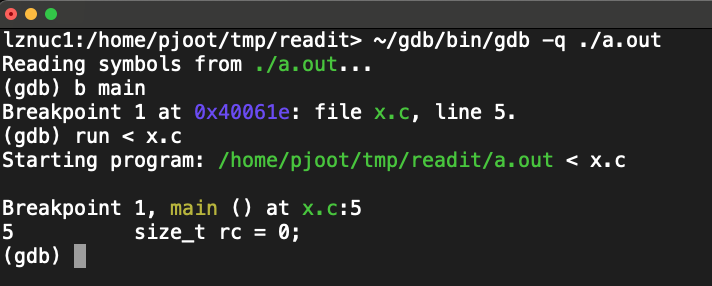I was asked how to let a gdb controlled slave read from stdin, and couldn’t remember how it was done, so I wrote the following little test program, figuring that muscle memory would remind me once I had gdb running.

#include <stdio.h>

int main()
{
size_t rc = 0;
char buf;

do {
rc = fread( buf, 1, 1, stdin );
if ( rc == 1 ) {
printf( "%c\n", buf );
}
} while ( rc );

return 0;
}


Turns out the answer is just the gdb run command, which can specify stdin for the program. Here’s a demo session that shows this in action

(gdb) b main
Breakpoint 1 at 0x40061e: file x.c, line 5.
(gdb) run < x.c

Breakpoint 1, main () at x.c:5
5          size_t rc = 0;
Missing separate debuginfos, use: yum debuginfo-install glibc-2.28-225.0.4.el8_8.6.x86_64
(gdb) n
9             rc = fread( buf, 1, 1, stdin );
(gdb) n
10            if ( rc == 1 ) {
(gdb) p rc
$1 = 1 (gdb) p buf$2 = 35 '#'
(gdb) n
11               printf( "%c\n", buf );
(gdb)
#
13         } while ( rc );
(gdb)
9             rc = fread( buf, 1, 1, stdin );
(gdb)
10            if ( rc == 1 ) {
(gdb) p rc
$3 = 1 (gdb) p buf$4 = 105 'i'


The x.c input that I passed to run, was the source of the program that I debugging.

## The evil of COBOL: everything is in global variables

COBOL does not have stack variables.  Everything is a global variable.  There is a loose equivalent of a function, called a paragraph, which can be called using a PERFORM statement, but a paragraph does not have any input or output variables, and no return code, so if you want it to behave like a function, you have to construct some sort of complicated naming convention using your global variables.

I’ve seen real customer COBOL programs with many thousands of global variables.  A production COBOL program is usually a giant sequence of MOVEs, MOVE A TO B, MOVE B TO C, MOVE C TO D, MOVE D TO E, … with various PERFORMs or GOTOs, or other things in between.  If you find that your variable has a bad value in it, that is probably because it has been copied from something that was copied from something, that was copied from something, that’s the output of something else, that was copied from something, 9 or 10 times.

I was toying around with the idea of coding up a COBOL implementation of 2D Euclidean geometric algebra, just as a joke, as it is surely the worst language in the world.  Yes, I work on a COBOL compiler project. The project is a lot of fun, and the people I work with are awesome, but I don’t have to like the language.

If I was to implement this simplest geometric algebra in COBOL, the logical starting place for that would be to implement complex numbers in COBOL first.  That is because we can use a pair of complex numbers to implement a 2D multivector, with one complex number for the vector part, and a complex number for the scalar and pseudoscalar parts.  That technique has been detailed on this blog previously, and also in a Mathematica module Cl20.m.

Trying to implement a couple of complex number operations in COBOL got absurd really fast.  Here’s an example.  First step was to create some complex number types.  I did that with a copybook (include file), like so: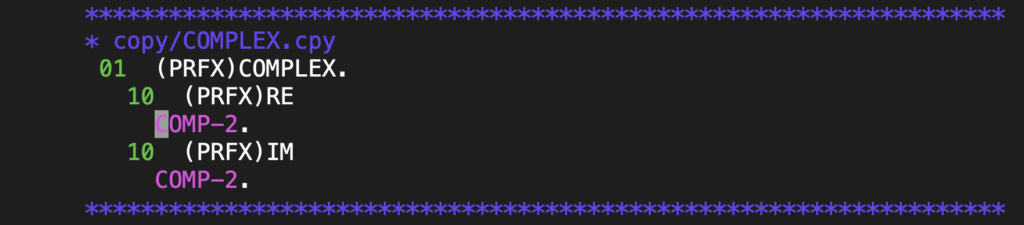This can be included multiple times, each time with a different name, like so: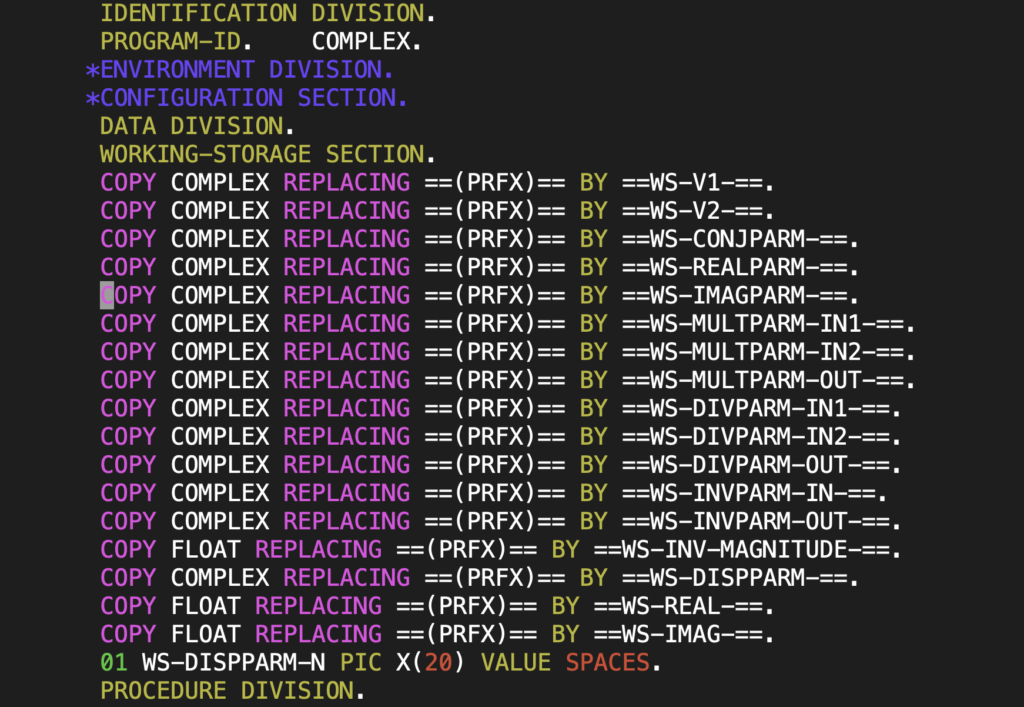The way that I structured all my helper functions, was with one set of global variables for input (at least one), and if appropriate, one output global variable.  Here’s an example: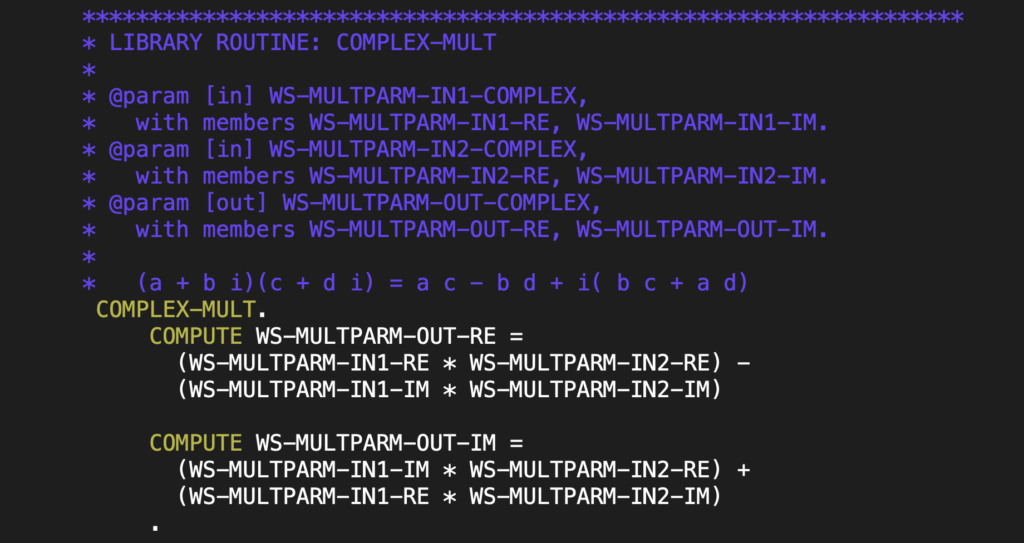So, if I want to compute and display a value, I have a whole pile of stupid MOVEs to do in and out of the appropriate global variables for each of the helper routines in question:I wrote enough of this little complex number library that I could do conjugate, real, imaginary, multiply, inverse, and divide operations.  I can run that little program with the following JCL

//COMPLEX JOB
//A EXEC PGM=COMPLEX
//SYSOUT   DD SYSOUT=*
//STEPLIB  DD DSN=PJOOT.SAMPLE.COMPLEX,
//  DISP=SHR


and get this SYSOUT:

STEP A SYSOUT:
A                    =  .10000000000000000E 01 + ( .20000000000000000E 01) I
B                    =  .30000000000000000E 01 + ( .40000000000000000E 01) I
CONJ(A)              =  .10000000000000000E 01 + (-.20000000000000000E 01) I
RE(A)                =  .10000000000000000E 01
IM(A)                =  .20000000000000000E 01
A * B                = -.50000000000000000E 01 + ( .10000000000000000E 02) I
1/A                  =  .20000000000000000E 00 + (-.40000000000000000E 00) I
A/B                  =  .44000000000000000E 00 + ( .80000000000000000E-01) I



If you would like your eyes burned further, you can access the full program on github here. It takes almost 200 lines of code to do almost nothing.